Home > CC4 > Chapter A > Lesson A.1.1 > ProblemA-8

A-8.

Read the Math Notes box for this lesson. For each of the following expressions, first indicate how many terms it has, and then combine like terms to simplify each algebraic expression, if possible. If it is not possible to simplify the expression, explain why not. Homework Help ✎

1. 3y + 2y + y2 + 5 + y

2. 3y2 + 2xy + 1 + 3x + y + 2x2

3. 3xy + 5x + 2 + 3y + x + 4

4. 4m + 2mn + m2 + m + 3m2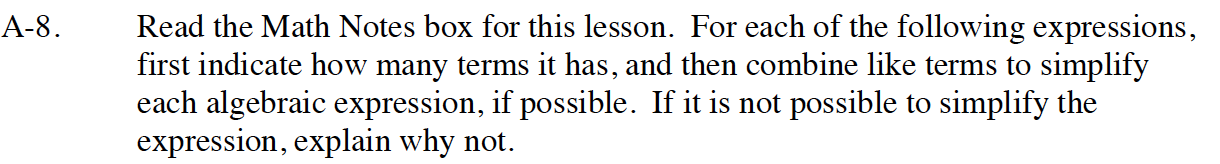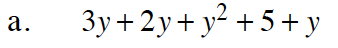Combine like terms.

3y + 2y + y + y² + 5

6y + y² + 5

Arrange from the highest to the lowest power.

y² + 6y + 5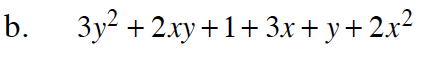Is there any way to combine terms?
Remember, you can only add terms if they have the exact same variable.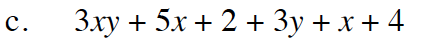Follow steps taken in part (a).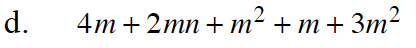Follow the steps taken in part (a).

4m² + 5m + 2mn